## 实现一个简单的解释器（5）

（已获得作者授权）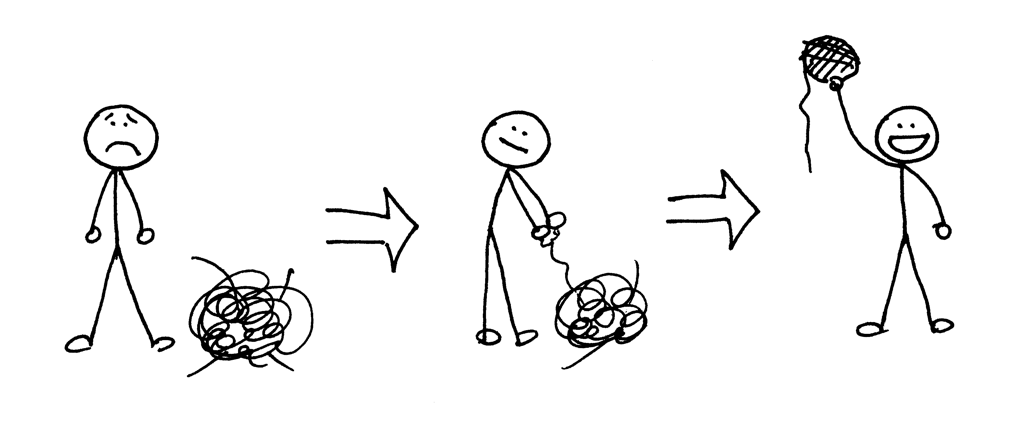7 + 3 + 1 is equivalent to (7 + 3) + 1
7 - 3 - 1 is equivalent to (7 - 3) - 1
8 * 4 * 2 is equivalent to (8 * 4) * 2
8 / 4 / 2 is equivalent to (8 / 4) / 2


7 + 3 - 1 is equivalent to (7 + 3) - 1
8 / 4 * 2 is equivalent to (8 / 4) * 2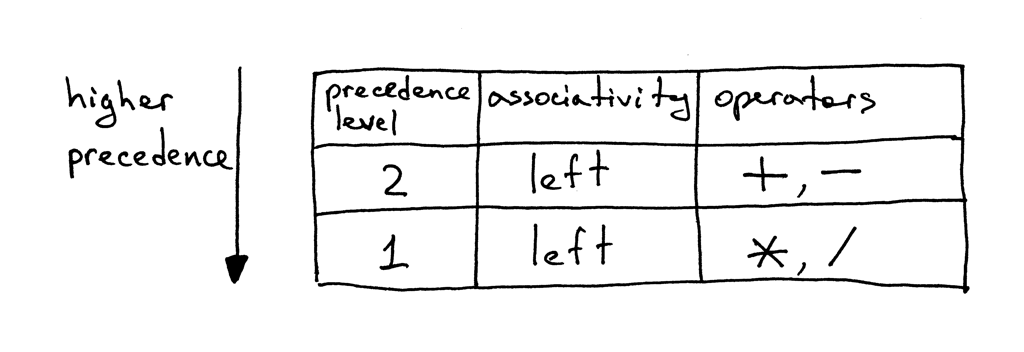1、为每一类优先级定义一个非终结符。非终极符的产生式主体应包含该类优先级的算术运算符和下一类更高优先级的非终结符。( The body of a production for the non-terminal should contain arithmetic operators from that level and non-terminals for the next higher level of precedence.)

2、为基本的表达单位（在本文下为整数）创建一个附加的非终结符factor。一般规则是，如果具有N类优先级，则总共将需要N + 1个非终结符：每类级别一个非终结符（N个）再加上一个运算基本单位的非终结符factor（1个）。(Create an additional non-terminal factor for basic units of expression, in our case, integers. The general rule is that if you have N levels of precedence, you will need N + 1 non-terminals in total: one non-terminal for each level plus one non-terminal for basic units of expression.)term产生式将包含一个使用级别1运算符的主题，即运算符乘号和除号，并且它也包含基本表达式单位（整数）的非终结符factor：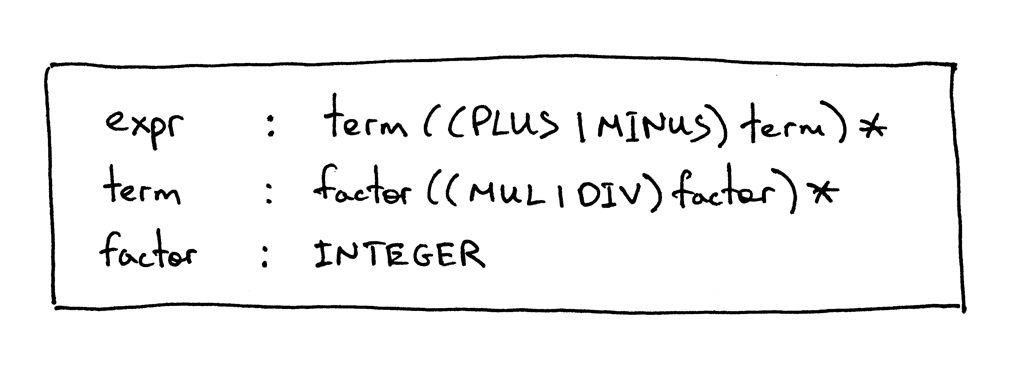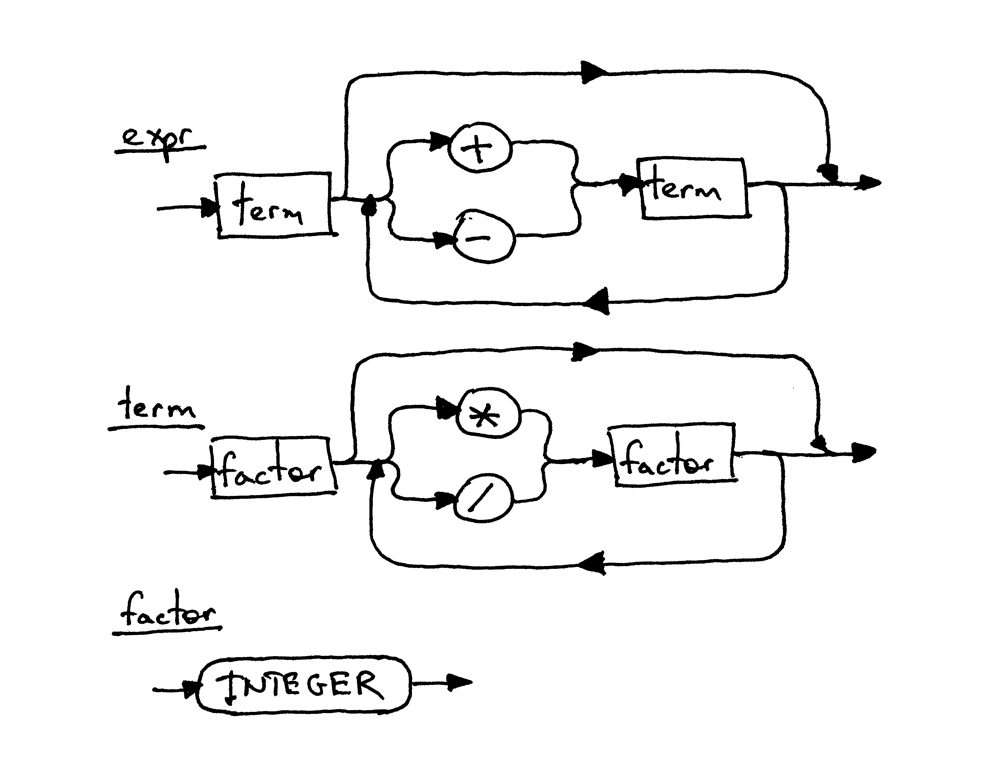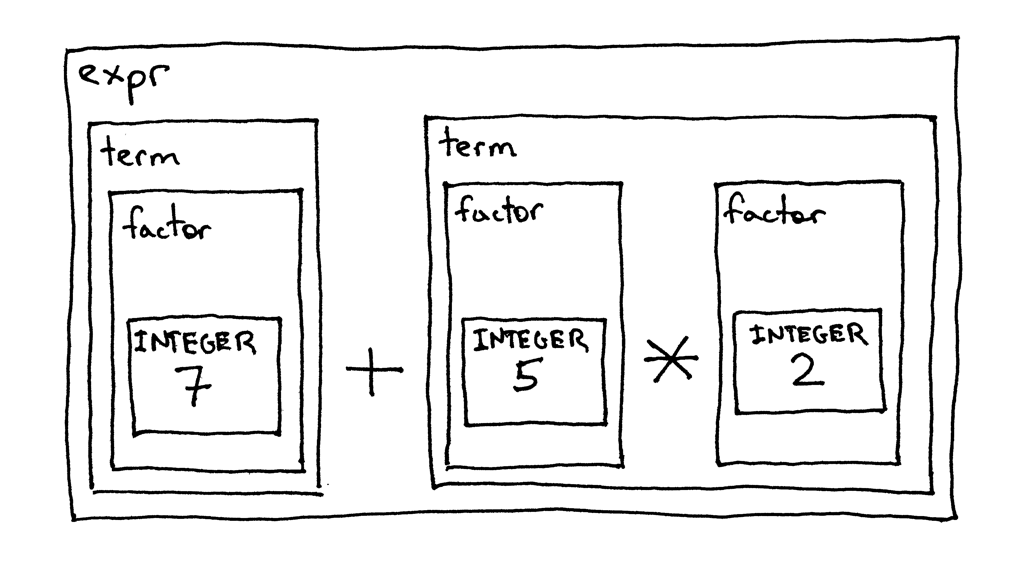1、Lexer类现在可以对+，-，*和/进行标记化（这里没有什么新鲜的，我们只是将以前文章中的代码组合到一个支持所有这些Token的类中）
2、回想一下，在语法中定义的每个规则（产生式）R都会转换为具有相同名称的函数，并且对该规则的引用会成为函数调用：R()， 所以Interpreter类现在具有对应于语法中三种非终结符函数：expr，term和factor。

# Token types
#
# EOF (end-of-file) token is used to indicate that
# there is no more input left for lexical analysis
INTEGER, PLUS, MINUS, MUL, DIV, EOF = (
'INTEGER', 'PLUS', 'MINUS', 'MUL', 'DIV', 'EOF'
)

class Token(object):
def __init__(self, type, value):
# token type: INTEGER, PLUS, MINUS, MUL, DIV, or EOF
self.type = type
# token value: non-negative integer value, '+', '-', '*', '/', or None
self.value = value

def __str__(self):
"""String representation of the class instance.

Examples:
Token(INTEGER, 3)
Token(PLUS, '+')
Token(MUL, '*')
"""
return 'Token({type}, {value})'.format(
type=self.type,
value=repr(self.value)
)

def __repr__(self):
return self.__str__()

class Lexer(object):
def __init__(self, text):
# client string input, e.g. "3 * 5", "12 / 3 * 4", etc
self.text = text
# self.pos is an index into self.text
self.pos = 0
self.current_char = self.text[self.pos]

def error(self):
raise Exception('Invalid character')

"""Advance the pos pointer and set the current_char variable."""
self.pos += 1
if self.pos > len(self.text) - 1:
self.current_char = None  # Indicates end of input
else:
self.current_char = self.text[self.pos]

def skip_whitespace(self):
while self.current_char is not None and self.current_char.isspace():

def integer(self):
"""Return a (multidigit) integer consumed from the input."""
result = ''
while self.current_char is not None and self.current_char.isdigit():
result += self.current_char
return int(result)

def get_next_token(self):
"""Lexical analyzer (also known as scanner or tokenizer)

This method is responsible for breaking a sentence
apart into tokens. One token at a time.
"""
while self.current_char is not None:

if self.current_char.isspace():
self.skip_whitespace()
continue

if self.current_char.isdigit():

if self.current_char == '+':

if self.current_char == '-':

if self.current_char == '*':

if self.current_char == '/':

self.error()

class Interpreter(object):
def __init__(self, lexer):
self.lexer = lexer
# set current token to the first token taken from the input
self.current_token = self.lexer.get_next_token()

def error(self):
raise Exception('Invalid syntax')

def eat(self, token_type):
# compare the current token type with the passed token
# type and if they match then "eat" the current token
# and assign the next token to the self.current_token,
# otherwise raise an exception.
if self.current_token.type == token_type:
self.current_token = self.lexer.get_next_token()
else:
self.error()

def factor(self):
"""factor : INTEGER"""
token = self.current_token
self.eat(INTEGER)

def term(self):
"""term : factor ((MUL | DIV) factor)*"""
result = self.factor()

while self.current_token.type in (MUL, DIV):
token = self.current_token
if token.type == MUL:
self.eat(MUL)
result = result * self.factor()
elif token.type == DIV:
self.eat(DIV)
result = result / self.factor()

return result

def expr(self):
"""Arithmetic expression parser / interpreter.

calc>  14 + 2 * 3 - 6 / 2
17

expr   : term ((PLUS | MINUS) term)*
term   : factor ((MUL | DIV) factor)*
factor : INTEGER
"""
result = self.term()

while self.current_token.type in (PLUS, MINUS):
token = self.current_token
if token.type == PLUS:
self.eat(PLUS)
result = result + self.term()
elif token.type == MINUS:
self.eat(MINUS)
result = result - self.term()

return result

def main():
while True:
try:
# To run under Python3 replace 'raw_input' call
# with 'input'
text = raw_input('calc> ')
except EOFError:
break
if not text:
continue
lexer = Lexer(text)
interpreter = Interpreter(lexer)
result = interpreter.expr()
print(result)

if __name__ == '__main__':
main()


\$ python calc5.py
calc> 3
3
calc> 2 + 7 * 4
30
calc> 7 - 8 / 4
5
calc> 14 + 2 * 3 - 6 / 2
171、在不浏览本文代码的情况下编写本文所述的解释器，完成后编写一些测试，并确保它们通过。

2、扩展解释器以处理包含括号的算术表达式，以便你的解释器可以计算深度嵌套的算术表达式，例如：7 + 3 *（10 /（12 /（3 + 1）-1））

1、运算符的左结合是什么意思？
2、运算符加号和减号是左结合还是右结合？ 乘号和除号呢？
3、运算符加号的优先级是否比运算符乘号高？

posted on 2020-03-03 16:36  Xlgd  阅读(...)  评论(...编辑  收藏

• 随笔 - 23
• 文章 - 0
• 评论 - 4